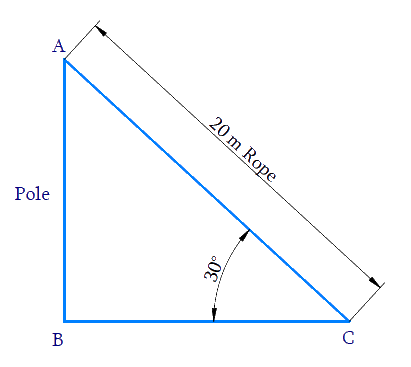# Ex. 9.1 Q1 Some Applications of Trigonometry Solution - NCERT Maths Class 10

Go back to  'Ex.9.1'

## Question

A circus artist is climbing a $$20\,\rm{m}$$ long rope, which is tightly stretched and tied from the top of a vertical pole to the ground. Find the height of the pole, if the angle made by the rope with the ground level is $$30^\circ$$ see Figure.

Video Solution
Some Applications Of Trigonometry
Ex 9.1 | Question 1

## Text Solution

What is Known?

(i) Length of rope $$=$$ $$20\,\rm{m}$$

(ii) Angle of rope with ground $$=$$ $$30^\circ$$$$=$$ $$\angle ACB$$

What is Unknown?

Height of poleReasoning:

$$AB =$$ Height of the Pole.

$$BC =$$ Distance between the point on the ground and the pole.

$$AC =$$ Length of the Rope (Hypotenuse).

We need to find the height of the pole $$AB,$$ from the angle $$C$$ and the length of the rope $$AC.$$ Therefore, Trigonometric ratio involving all the three measures is $${\rm{sin}}\, C.$$

In $$\Delta ABC$$,

\begin{align} {AB} &=\frac{\text { opposite }}{\text { hypotenuse }} \\ {\rm{sin}}\,C &=\frac{{AB}}{{AC}} \\ {\rm{sin}}\, {C} &=\frac{{AB}}{20} \\ \frac{1}{2} &=\frac{{AB}}{20} \\ {AB}&=\frac{1}{\not{2}} \times \not\!\!{20}^{10}\ \\ {AB} &=10 \rm {m} \end{align}

Height of pole $$AB = 10\rm\,m.$$

Learn from the best math teachers and top your exams

• Live one on one classroom and doubt clearing
• Practice worksheets in and after class for conceptual clarity
• Personalized curriculum to keep up with school